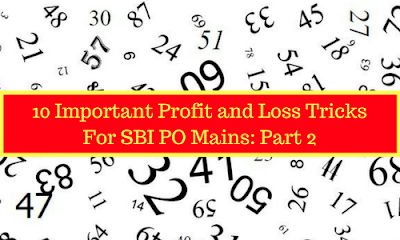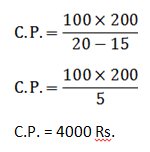Gurpurab Offer - Use Code GURPURAB2023

# 10 Important Profit and Loss Tricks: Part 2### Trick 1.

If the selling price of two items is same but one item is sold at x% profit and another is sold at x% loss, then loss %
(In this case, there is always a loss.)

Example

If Ram sold two T.V. in the same price. He sold one T.V. at 10% profit and another at 10% loss. Find the % loss/ profit on total.
If a shopkeeper sells his goods at cost price but uses a weight of ‘x’ gram instead of ‘y’ gram to gain ‘a%’ profit
A dishonest dealer profess to sell his goods at C.P. and uses a weight of 875 gram instead of 1 kg. to gain X% profit. Find the value of X.

If a shopkeeper sells his goods at ‘x%’ loss on cost price but uses ‘y’ gm instead of ‘z’ gm, then, his % profit or loss
A dishonest dealer sells goods at 25/4% loss on cost price but uses the weight of 14 gram instead of 16 gram. What is his % profit or loss?
A person sells an item at ‘x%’ profit. If he sells it for ‘A’ Rs. more, he would make a profit of ‘y%’, then

A person sells a bag at 15% profit. If he sells it for 200 Rs. more, he would make a profit of 20, then find the C.P.
Solution:C.P. = 4000 Rs.

### Trick  5.

A person sells an item at ‘x%’ profit. If he sells it for ‘A’ Rs. Less, he would make a loss of ‘y%’, then

A person sold his table at 15% profit. If he sold it for 500 Rs. less, than he had a loss of 10%. What is the C.P. of that table?
Solution:C.P. = 2000 Rs.

### Trick 6.

A person sells an item at ‘x%’ loss. If he sells it for ‘A’ Rs. more, he would make a loss of ‘y%’, then
Mahesh sold a Phone at a loss of 10%. If he had sold it at 5% loss than he have got 60 Rs. more. What is C.P. of that phone?
If on selling an item for ‘X’ Rs., a person gets profit equal to the loss if he sells it for ‘Y’ Rs., than cost price of that item will be
Ajay sells an item for 2540 Rs. and gets profit equal to the loss if he sells it for 1850 Rs. than find out the C.P. of that item.
Solution:
If a man buys 'p' articles for 'a' rupees and sells 'q' articles for 'b' rupees. Then,
If Sheena buys 4 Toffees for 5 Rs. and sells 5 Toffee for 4 Rs. Then what is profit/loss %?
(Sign is -ive)

% loss = 36%

### Trick  9.

If the successive discounts given on a product are p%, q% and r%, then the selling price after all the discounts is:

After a successive discount of 10% and 8%, an article was sold for Rs. 4968. What is the M.R.P. of that article?
Solution:M.R.P. = 6000 Rs.

### Trick 10.

If the selling price of 'X' pens is equal to the cost price of Y' pens. Then

If the selling price of 60 eggs is equal to the cost price of 75 eggs. What is the profit % of that eggs?Profit % = 25%